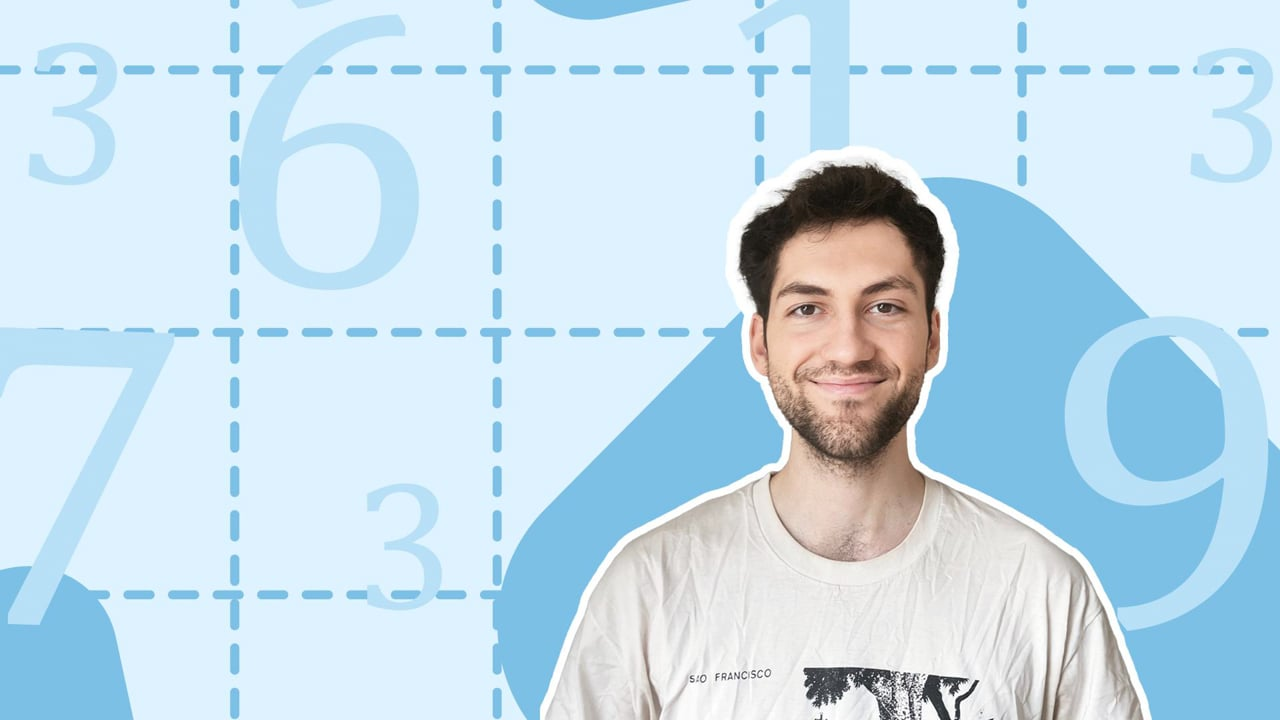Chapter overviewMaths

Number and place value

Multiplication and division

Fractions

Measurement

Geometry - properties of shapes

Statistics

Maths

# Subtracting numbers using mental maths0%

Summary

# Subtracting numbers using mental maths

## ​​In a nutshell

By keeping track of your units, tens, hundreds, or other place values with many digits, you can subtract numbers using mental maths.

## Subtracting a small number

Subtracting is just counting down. So $6-4$ is just counting backwards from six by four. If you know how to count backwards, you know how to subtract.

##### Example 1

Calculate

$8-3$​​

This is the same as counting backwards from eight by three.

So

$8-3=\underline{5}$

## ​​​​Subtracting a larger number

For larger numbers, counting in jumps of one will take too long. Instead, you break down the number you are subtracting into its units, tens, hundreds, and other place values, and count those jumps individually.

##### Example 2

Calculate

$97-35$​​

Start by subtracting the units. There are five units to subtract. Next, subtract the three tens.

​​

Hence

$97-35=\underline{62}$​​

## Changing other place values

Often when subtracting, you may be subtracting some units only for example, but will have to change the tens value. This can be true of any place value: you may be subtracting thousands, but have to change the tens of thousands value.

##### Example 3

Calculate

$215-76$​​

Start by subtracting the six units. Notice that the tens unit has dropped by one even though you were subtracting the units. Next, subtract the seven tens from $209$:

Notice that when subtracting the tens, you have changed the hundreds unit. This is because you subtracted to go below $0$ in the tens column. Thus

$215-76=\underline{139}$

## Want to find out more? Check out these other lessons!

FAQs

• Question: What is subtraction?

Answer: Subtracting is just counting down. It is reducing the value of your number. So 6−4 is just counting backwards from six by four. If you know how to count backwards, you know how to subtract.

• Question: How can you subtract mentally?

Answer: Imagine a number line and count backwards by the number you are subtracting.

Theory

Exercises